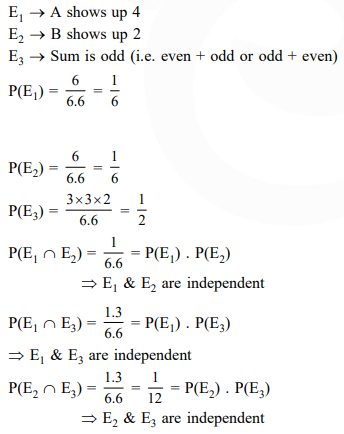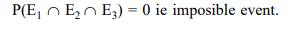# Let two fair six-faced dice

Question:

Let two fair six-faced dice $\mathrm{A}$ and $\mathrm{B}$ be thrown simultaneously. If $\mathrm{E}_{1}$ is the event that die A shows up four, $E_{2}$ is the event that die $B$ shows up two and $E_{3}$ is the event that the sum of numbers on both dice is odd, then which of the following statements is NOT true?

1. $\mathrm{E}_{1}, \mathrm{E}_{2}$ and $\mathrm{E}_{3}$ are independent.

2. $\mathrm{E}_{1}$ and $\mathrm{E}_{2}$ are independent.

3. $\mathrm{E}_{2}$ and $\mathrm{E}_{3}$ are independent.

4. $\mathrm{E}_{1}$ and $\mathrm{E}_{3}$ are independent.

Correct Option: 1

Solution: